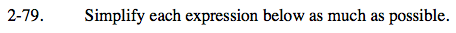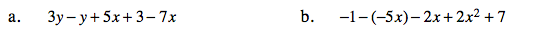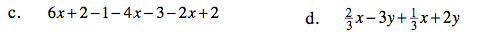### Home > CAAC > Chapter 2 > Lesson 2.1.8 > Problem2-79

2-79.
1. Simplify each expression below as much as possible. Homework Help ✎

1. 3y − y + 5x + 3 − 7x

2. −1 − (−5x) − 2x + 2x2 + 7

3. 6x + 2 − 1 − 4x − 3 − 2x + 2

4.Try using an Expression Mat.
3y + (−y) + 5x + 3 + (−7x)

Combine like terms.
2y + (−2x) +3

Remember to remove unnecessary signs.
(Example: x + (−2) would be x −2

2y −2x +3

Follow the steps described in part (a).

Remember you cannot add variables with different exponent values. (Example: you cannot add x + x2)

2x 2 + 3x +6$\frac{2}{3}x+\frac{1}{3}x=x$